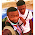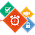# Program To Count Number Of Negative, Positive And Zeros Input

The program below is the answer to Deitel's Java How to Program (9th Edition) Chapter 2 Exercise 2.32.

Question: Write a program that inputs five numbers and determines and prints the number of negative numbers input, the number of positive numbers input and the number of zeros input.

```/**
*
* @Author: Aghatise Osazuwa
* Website: www.cscprogrammingtutorials.com
*
* Exercise 2.32 - Negative, Positive And Zero Values
* This Program Displays The Number Of Negative, Positive And Zero Values From Five Numbers
*
*/

import java.util.Scanner;

public class Ex02_32 {
public static void main (String [] args) {

Scanner value = new Scanner (System.in);

int num1;
int num2;
int num3;
int num4;
int num5;
int numPositive = 0; //variable to count how many positive numbers initialized to zero
int numZero = 0; //variable to count how many numbers are zero initialized to zero
int numNegative = 0; //variable to count how many negative numbers initialized to zero

System.out.print ("Enter Your First Number: ");
num1 = value.nextInt();
System.out.print ("Enter Your Second Number: ");
num2 = value.nextInt();
System.out.print ("Enter Your Third Number: ");
num3 = value.nextInt();
System.out.print ("Enter Your Fourth Number: ");
num4 = value.nextInt();
System.out.print ("Enter Your Fifth Number: ");
num5 = value.nextInt();

//count negative numbers

if (num1 < 0)
numNegative = numNegative + 1;
if (num2 < 0)
numNegative = numNegative + 1;
if (num3 < 0)
numNegative = numNegative + 1;
if (num4 < 0)
numNegative = numNegative + 1;
if (num5 < 0)
numNegative = numNegative + 1;

//count positive numbers

if (num1 > 0)
numPositive = numPositive + 1;
if (num2 > 0)
numPositive = numPositive + 1;
if (num3 > 0)
numPositive = numPositive + 1;
if (num4 > 0)
numPositive = numPositive + 1;
if (num5 > 0)
numPositive = numPositive + 1;

//count number of zeros

if (num1 == 0)
numZero = numZero + 1;
if (num2 == 0)
numZero = numZero + 1;
if (num3 == 0)
numZero = numZero + 1;
if (num4 == 0)
numZero = numZero + 1;
if (num5 == 0)
numZero = numZero + 1;

System.out.println ("\n");

System.out.printf ("Negative numbers = %d\n", numNegative);
System.out.printf ("Positive numbers = %d\n", numPositive);
System.out.printf ("Zeros = %d\n", numZero);

}
}
```

Click here to see other answers to Java How to Program.

1.please send the flow use diagram and the algorithm for this program, its for academic assignment.....

2.how about using an array(i) of a given size for input then compare the numbers entered. wont that shorten the program?

1.this chapter doesnt include arrays

DISCLAIMER: Opinions expressed in comments are those of the comment writers alone and does not reflect or represent the views of the post author. We reserve the right to delete any post deemed inappropriate or offensive and/or spammy. Please do not use abusive words/hate speech.

I Appreciate your valuable Feedback. So, Please DO NOT SPAM - Spam comments will be deleted immediately.

Don't use brand name in name field and you're not allowed to use links in comments unless it's necessary. Such comments will be removed immediately. To get notified of replies or follow-up comments, click the box next to notify me.

Thanks.